×

Search anything:

#### Data Structures Algorithms linked listGet this book -> Problems on Array: For Interviews and Competitive Programming

In this article, we have explored Circular Doubly Linked List and operations that can be performed on it. It is a combination to two Data Structures namely Circular Linked List and Doubly Linked List.

1. Introduction to Circular Doubly Linked List
2. Insertion at the beginning
3. Insertion at the end
4. Insertion at a given position
5. Deletion at the beginning
6. Deletion at the end
7. Deletion at a given position
8. Traverse
9. Search
10. Applications

Pre-requisites:

## 1) Introduction to Circular Doubly Linked List

Circular Doubly Linked List is a linear data structure which consists of nodes. Each node is divided into three parts containing data part,pointer to previous node and pointer to next node.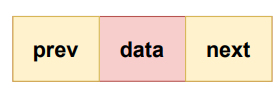Unlike Linked List or Doubly Linked List, Circular Doubly Linked List doesn't contain NULL values in any of the nodes rather the head's previous pointer points to last node and last node's next pointer points to head. This is an advantage as NULL is considered as a special case.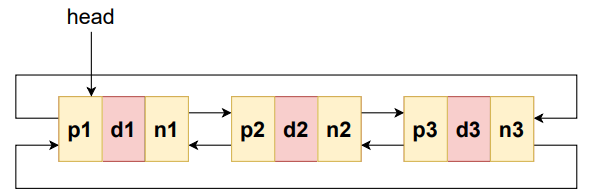A Circular Doubly Linked List consisting of three nodes

Node structure:

``````class ListNode:
def __init__(self,value):
self.prev=None
self.data=value
self.next=None
``````

Various operations can be performed on Circular Doubly Linked List as discussed below.

### Insertion at the beginning

In this operation a new node is inserted at the beginning of CDLL.

The basic idea is to update the head node of Circular Doubly Linked List which is the starting point and the rest of the process remain the same as in inserting in any other position.

Note: As any node in Circular Doubly Linked List can act as the head node, it is important to maintain it but it does not create issues if access to head node is lost provided we have access to any node of the Circular Doubly Linked List.

Let's understand through an illustration.

Given CDLL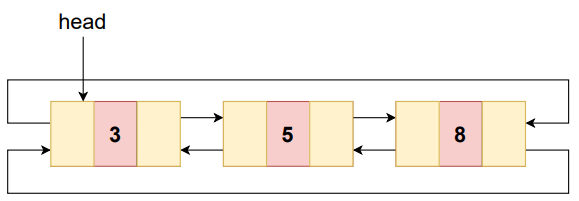Create a new node with value 2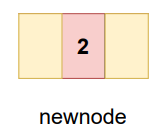Take a variable ptr and let it point to head initially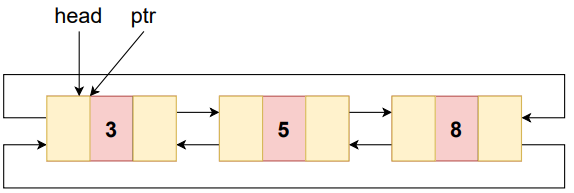Traverse through the list to make ptr point to the last node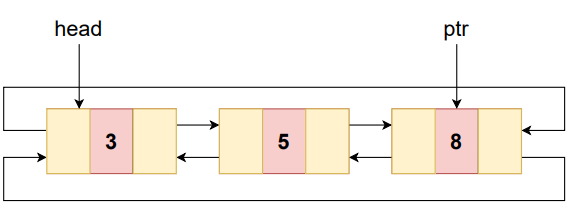Change the pointers as follows

ptr->next=newnode
-Last node's next pointer will point to new node as it'll be new head.
newnode->prev=ptr
-New node's prev pointer will point to ptr as head's previous must be last node.
-New node's next pointer will point to current head as current head'll become the second node.
-Current head's prev pointer will point to new node as it'll be the new head now.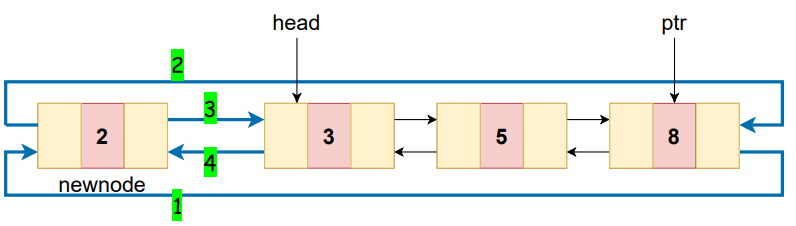-Finally head pointer will point to the new node.

-The modified list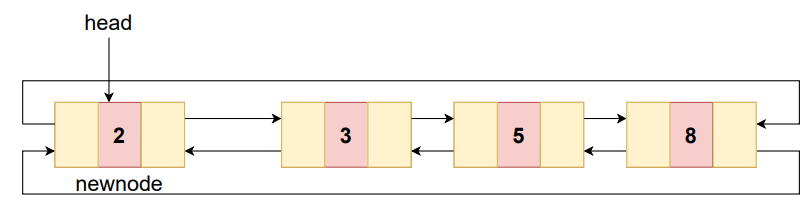### ALGORITHM

STEP-1: Create newnode
STEP-4:  SET ptr=ptr->next
[END OF LOOP]
STEP-5: SET ptr->next=newnode
STEP-6: SET newnode->prev=ptr
STEP-10: EXIT

### CODE SNIPPET

``````def insertAtBeginning(value):
newnode=ListNode(value)
return
ptr=ptr.next
ptr.next=newnode
newnode.prev=ptr
``````

## Insertion at the end

In this operation a new node is inserted at the end of CDLL.

Similar to the previous case, the head is modified in this case as well as the last node points to the head node through the next pointer.

Let's understand through an illustration.

Given CDLL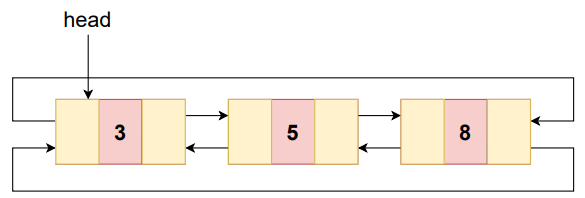Create a new node with value 2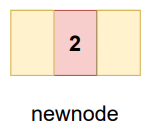Take a variable ptr and let it point to head initially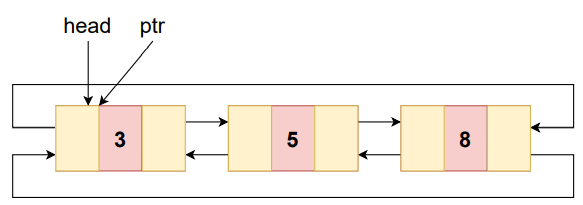Traverse through the list to make ptr point to the last node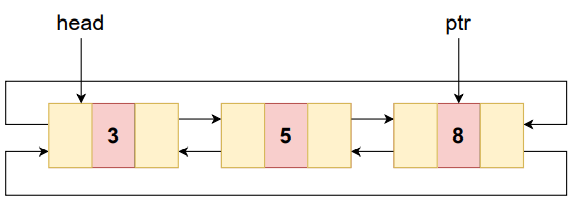Change the pointers as follows

-New node's next pointer will point to head as it'll become the last node.
ptr->next=newnode
-Current last node's next pointer will point to new node as it'll become the second last node now.
newnode->prev=ptr
-New node's prev pointer will point to current last node.
-Head's prev pointer will point to new node as new node will become the last node.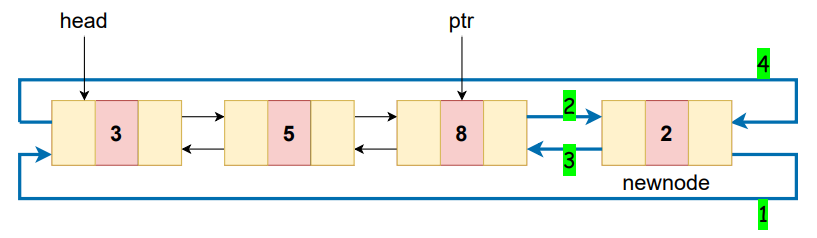The modified list.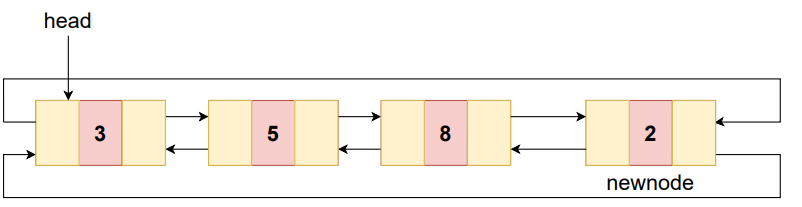#### ALGORITHM

STEP-1: Create newnode
STEP-4:  SET ptr=ptr->next
[END OF LOOP]
STEP-6: SET ptr->next=newnode
STEP-7: SET newnode->prev=ptr
STEP-9: EXIT

### CODE SNIPPET

``````def insertAtEnd(value):
newnode=ListNode(value)
return
ptr=ptr.next
ptr.next=newnode
newnode.prev=ptr
``````

### Insertion at a given position

In this operation a new node is inserted at a given position of CDLL.
Let's understand through an illustration.

Given CDLL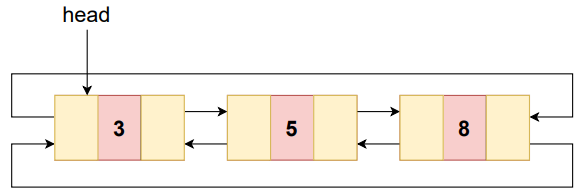Create a new node with value 2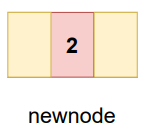Let the new node be inserted at pos=3

Take a variable ptr and let it point to head initially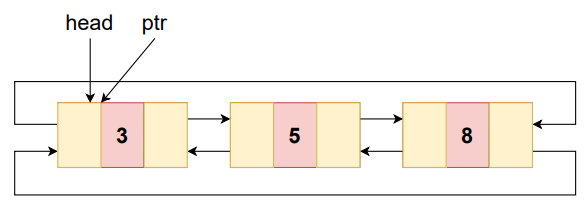Traverse through the list to make ptr point to the node with position pos-1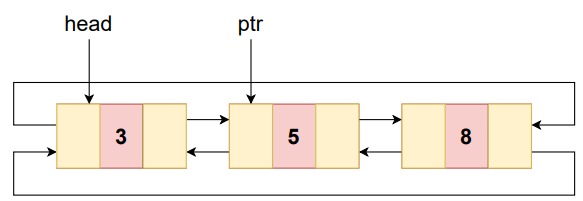Change the pointers as follows

newnode->next=ptr->next
-new node's next pointer will point to ptr's next
newnode->prev=ptr
-new node's prev pointer will point to ptr as ptr will be before new node.
ptr->next->prev=newnode
-ptr's next i,e., the node which will be after new node.Its prev pointer will point to new node as new node will be before it.
ptr->next=newnode
-ptr's prev pointer will point to new node as new node will be after it.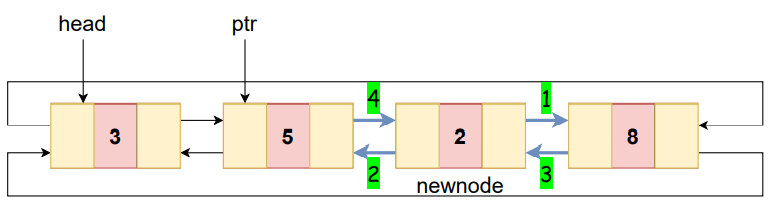The modified list.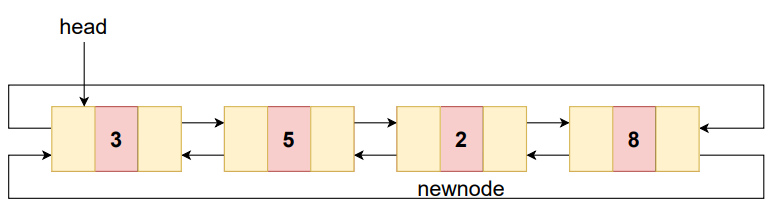#### ALGORITHM

STEP-1: Create newnode
STEP-3: SET i=1
STEP-4: Repeat STEPS-5 to 6 while i!=pos-1
STEP-5:  SET ptr=ptr->next
STEP-6:  SET i=i+1
[END OF LOOP]
STEP-7: SET newnode->next=ptr->next
STEP-8: SET newnode->prev=ptr
STEP-9: SET ptr->next->prev=newnode
STEP-10: SET ptr->next=newnode
STEP-11: EXIT

### CODE SNIPPET

``````def insertAtGivenPos(pos,value):
newnode=ListNode(value)
return
i=1
while i!=pos-1:
ptr=ptr.next
i+=1
newnode.next=ptr.next
newnode.prev=ptr
ptr.next.prev=newnode
ptr.next=newnode
``````

## Deletion at the beginning

In this operation the node at the beginning i.e., node to which head is pointing is deleted.
Let's understand through an illustration.

Given CDLL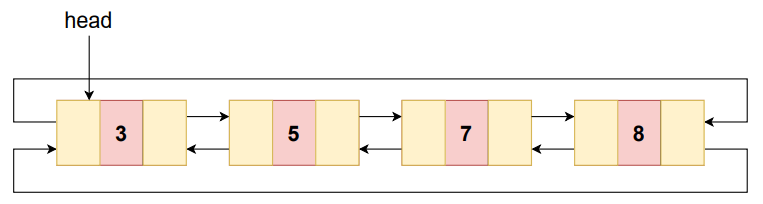Node to be deleted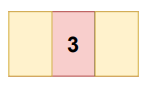Take a variable ptr and let it point to head initially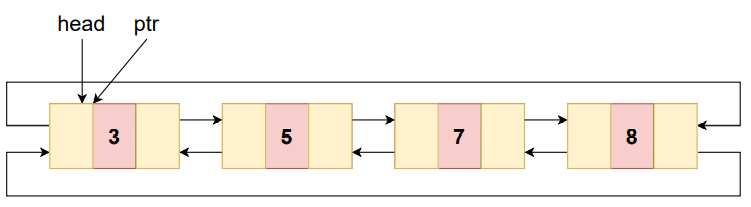Traverse through the list to make ptr point to the last node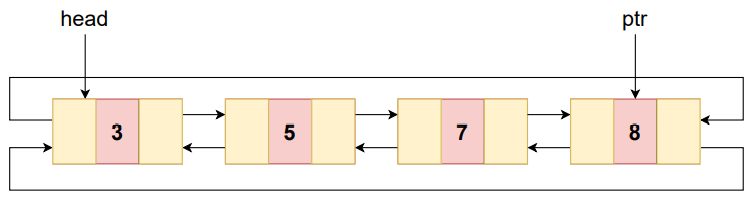Change the pointers as follows

-last node's next pointer will point to head's next as current head's next will become the new head
-prev pointer of head's next, which will become the new head ,will point to last node.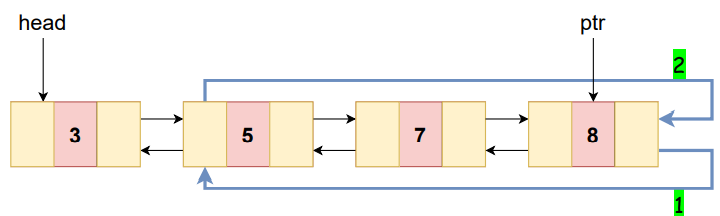-Free the memory allocated to current head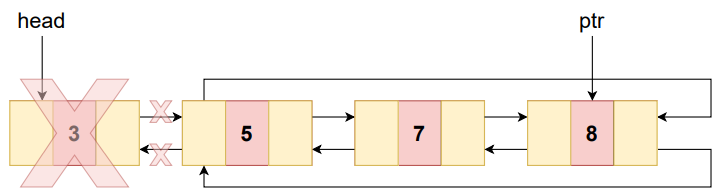-Change head to ptr's next as it points to the new head

The modified list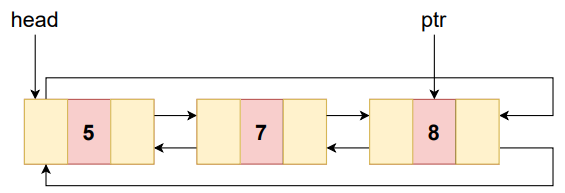#### ALGORITHM

STEP-3:  SET ptr=ptr->next
[END OF LOOP]
STEP-8: EXIT

### CODE SNIPPET

``````def deleteAtBeginning():
print("Deletion not possible")
return
ptr=ptr.next
``````

## Deletion at the end

In this operation the node at the end i.e., the last node of CDLL is deleted.
Let's understand through an illustration.

Given CDLL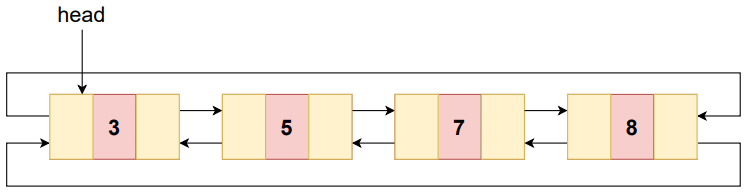Node to be deleted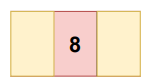Take a variable ptr and let it point to head initially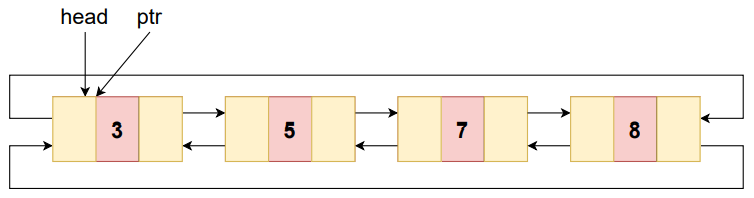Traverse through the list to make ptr point to the last node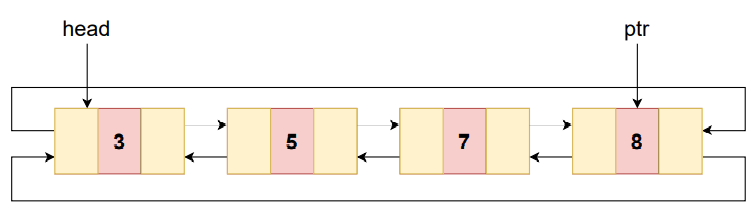Change the pointers as follows

-next pointer of last node's prev will point to head as last node will become the last node.
-ptr's prev node will become the last node.Hence, head's prev pointer will point to it.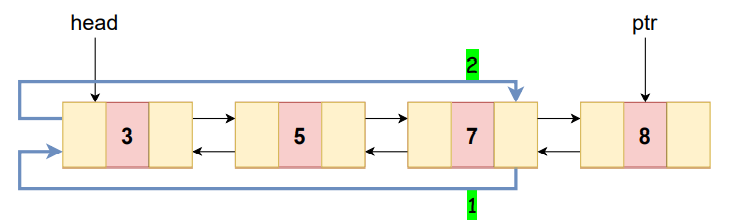free(ptr)
-Free the memory allocated to current last node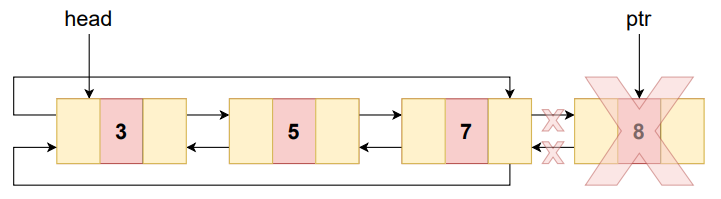The modified list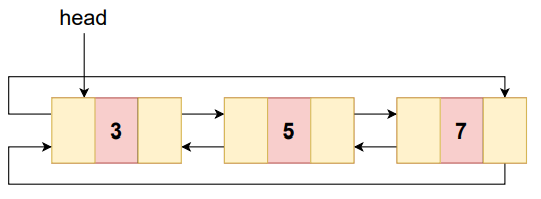#### ALGORITHM

STEP-3:  SET ptr=ptr->next
[END OF LOOP]
STEP-6: free(ptr)
STEP-7: EXIT

### CODE SNIPPET

``````def deleteAtEnd():
print("Deletion not possible")
return
ptr=ptr.next
del ptr
``````

### Deletion at a given position

In this operation the node at a given position in CDLL is deleted.
Let's understand through an illustration.

Given CDLL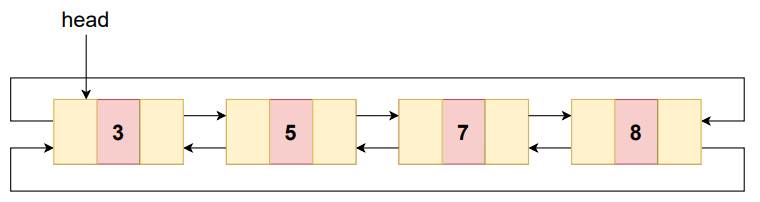Node to be deleted at position 3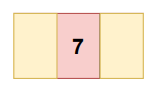Take a variable ptr and let it point to head initially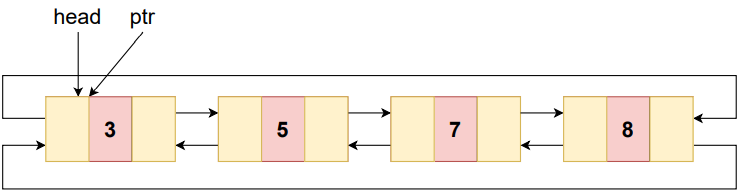Traverse through the list to make ptr point to the node with position pos-1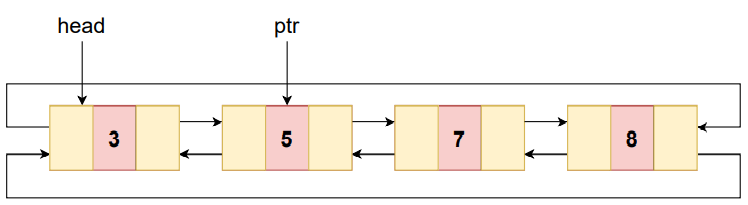Make a variable temp to point to ptr's next i.e., the node to be deleted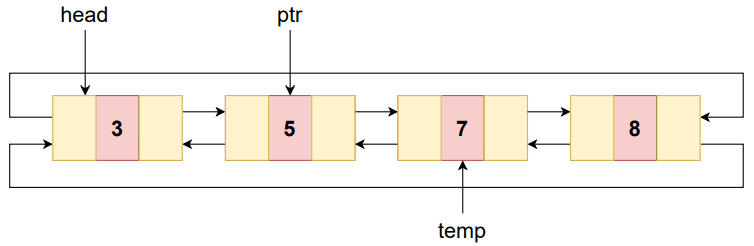Change the pointers as follows

temp->next->prev=ptr
-prev pointer of temp's next will point to ptr
ptr->next=temp->next
-ptr's next pointer will point to temp's next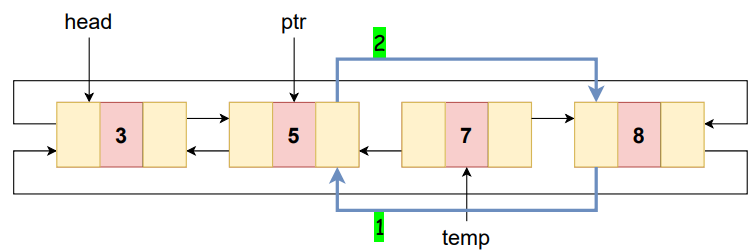free(temp)
-Free the memory allocated to node pointed by temp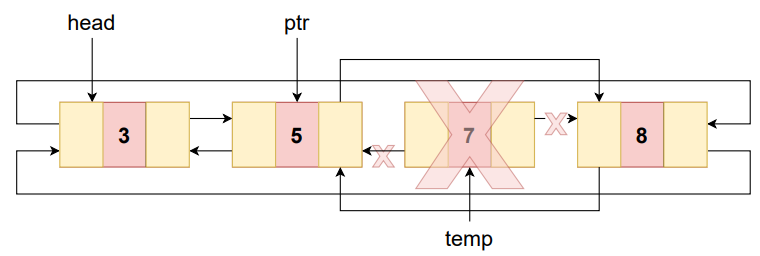-The modified list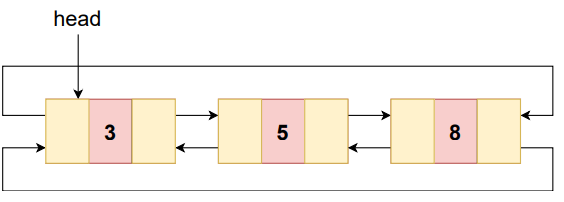#### ALGORITHM

STEP-2: SET i=1
STEP-3: Repeat STEPS-4 to 5 while i!=pos-1
STEP-4:  SET ptr=ptr->next
STEP-5:  SET i=i+1
[END OF LOOP]
STEP-6: SET temp=ptr->next
STEP-7: SET temp->next->prev=ptr
STEP-8: SET ptr->next=temp->next
STEP-9: free(temp)
STEP-10: EXIT

### CODE SNIPPET

``````def deleteAtGivenPos(pos):
print("Deletion not possible")
return
i=1
while i!=pos-1:
ptr=ptr.next
i+=1
temp=ptr.next
temp.next.prev=ptr
ptr.next=temp.next
del temp
``````

## Traverse

Traversing a data structure means to visit each of its elements exactly once.
In this operation each node of the CDLL is visited.
Let's understand through an illustration.

Given CDLL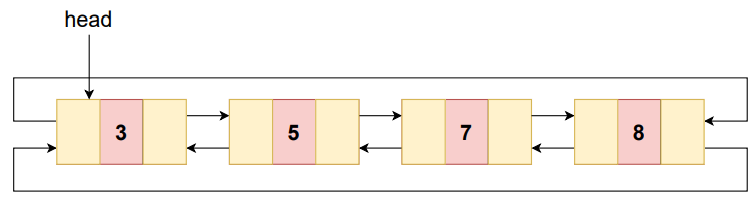Take a variable ptr and let it point to head initially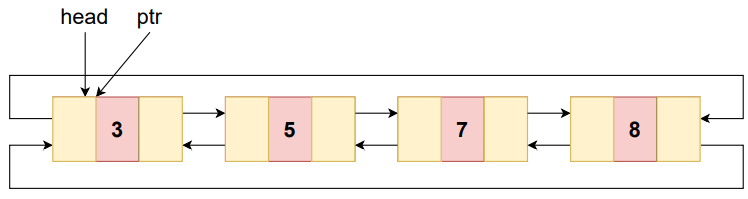Traverse through the list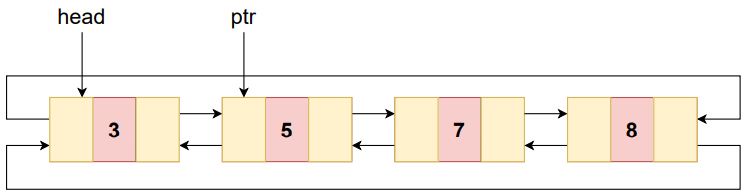Traverse until ptr points to the last node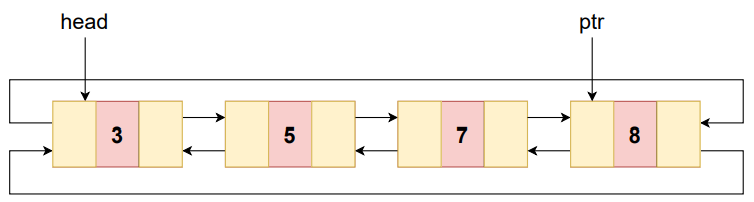When visited print each node's value

Result: 3 5 7 8

#### ALGORITHM

STEP-2: Repeat STEPS-3 to 4 while ptr->next!=head
STEP-3:  PRINT ptr->data
STEP-4:  SET ptr=ptr->next
[END OF LOOP]
STEP-5: EXIT

### CODE SNIPPET

``````def traverse():
print("List is empty!")
return
print(ptr.data)
ptr=ptr.next
``````

Search operation is used to know whether an element with a given value exists or not in the data structure.
In this operation a target value is searched.
Let's understand through an illustration.

Given CDLL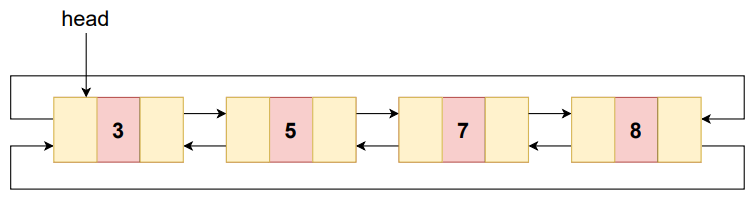Let the target to be searched is 5

Take a variable ptr and let it point to head initially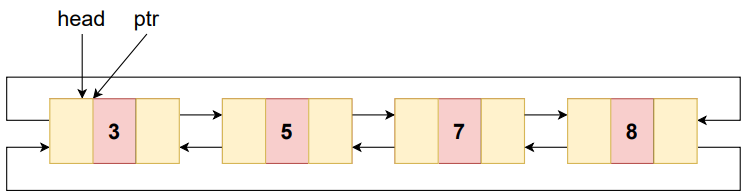While traversing if the node's value is target then search is successful
Here 5 is found hence the search is successful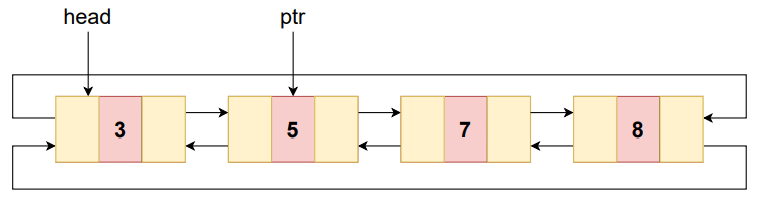After traversing the entire list if none of the node's value is target then search is unsuccessful

#### ALGORITHM

STEP-2: SET found=false
STEP-3: Repeat STEPS-4 to 8 while ptr->next!=head
STEP-4:  IF ptr->data=target
STEP-5:   PRINT ptr->data
STEP-6:   found=true
STEP-7:   Go to STEP-9
[END OF IF]
STEP-8:  SET ptr=ptr->next
[END OF LOOP]
STEP-9: IF found=true
PRINT "target found!"
STEP-10: ELSE
[END OF IF]
STEP-11: EXIT

### CODE SNIPPET

``````def search(target):
found=False
if ptr.data==target:
found=True
break
ptr=ptr.next
if found==True:
print("target found!")
else:
``````

## 3)CONCLUSION

Let n be the size of Circular Doubly Linked List.
Each of the above discussed operations takes O(n) time complexity.

## 4) Applications

Applications of Circular Doubly Linked List are:

-Managing songs playlist in media player applications.
-Managing shopping cart in online shopping.